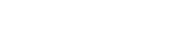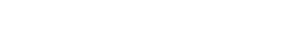# Determine Water Content of Soil by Oven Dry and Pycnometer MethodsReading time: 1 minute

## What is Water Content of Soil?

The water content of soil is is the ratio of mass of water to mass of soil which is expressed in percentage. Oven Dry Method and Pycnometer Method are commonly used to determine the water content of soil in laboratory.

## 1. Water Content of Soil by Oven Dry Method

The oven dry method is widely used laboratory method determine the water content or moisture content of given soil sample. It gives very accurate results.

### Apparatus Required for Oven Dry Method

To determine water content by oven dry method following equipment is required.
• Hot Air Oven
• Non-corrodible air tight containers ( 3 no’s)
• Digital Weight Machine (accuracy of 0.04% of mass of sample)
• Desiccator
• Tongs

### Minimum Soil Sample Quantity

The soil sample collected from the field should be of required quantity to find the water content. The quantity of soil required is depends upon the maximum particle size and gradation of soil sample. Below table shows the soil quantity required for test based on the sieve analysis.

Table 1: Minimum Quantity of Soil Required for Water Content Determination

 Size of particles more than 90% of passing Minimum Quantity ( grams) 425 micron sieve 25 2 mm sieve 50 4.25 mm sieve 200 10 mm sieve 300 20 mm sieve 500 40 mm sieve 1000Fig 1: Wet Soil Sample

### Test Procedure of Oven Dry Method

The test procedure of Oven Dry method to find the moisture content of soil consists following steps.
• In first step, clean and dry the containers and weigh them and note down the mass of each container (M1). Also note down the number of each container along with its weight.
• Collect the soil sample from field. Remove the top layer of soil and collect the wet soil from bottom layers.
• Fill the containers with required quantity of soil sample and weigh the each container and note down its mass (M2).Fig 2: Placing Containers in Oven

• Place the containers in hot air oven, arrange temperature to 110o ± 5o C and allow them to dry for 24 hours.
• After 24 hours turn off the oven and take out the containers using tongs.
• Cool down the containers in desiccator for one hour.
• After that weigh containers and note down the mass (m3) of each container.

### Observations and Calculations of Oven Dry Method

The data collected during the test is noted in below data sheet. From this data the water content of given soil sample is calculated by the below shown formulaWhere     M1= Mass of empty container with lid, M2= Mass of the container with wet soil and lid, M3= Mass of the container with dry soil and lid. Table 2: Observations and Calculations of Oven Dry Method
 Sl. No. Observations and Calculations Determination No. 1 2 3 Observation 1 Container No. 2 Mass of empty container (M1) 3 Mass of container + soil (M2) 4 Mass of container + dry soil (M3) Calculations 5 Mass of water Mw= M2 – M3 6 Mass of solids, Ms= M3 – M1 7 Water content= (5)/(6)x100

### Result of Oven Dry Method

Water content of the given soil sample = ______%.

## 2. Water Content of Soil by Pycnometer Method

Pycnometer method is also useful to determine water content. But this is used when the specific gravity of the given soil sample is already known. However, Specific gravity can also be determined using pycnometer. Read also: Specific Gravity of Soil by Pycnometer Method

### Apparatus Required for Pycnometer Method

Apparatus used in pycnometer method are
• Pycnometer
• Weighing balance with an accuracy of 1.0g
• Glass rod
• Vacuum pumpFig 3: Pycnometer Bottle

### Test Procedure of Pycnometer Method

Test procedure of pycnometer method is as follows
• Wash, clean and dry the pycnometer and note down its mass (M1) along with brass cap and washer using weighing balance with an accuracy of 1.0 g.
• Now place a sample of wet soil about 200 to 400 g in pycnometer and note down its mass (M2).
• Then add water to the soil in the pycnometer to make it about half full.
• Stir the soil using glass rod to remove air voids of the soil sample. If available connect the vacuum pump to the soil specimen to remove entrapped air.
• Add some more water and after eliminating the entrapped air stop stirring and fix the brass cap. More water is added through hole in brass cap until the water is flush with the hole.
• Now take the mass of pycnometer (M3).
• Now empty and wash the pycnometer. Then fill it with only water and take its mass (M4).

### Observations and Calculations of Pycnometer Method

The water content (w) of the soil sample using pycnometer method is calculated from the below formulaWhere   M1=mass of empty Pycnometer, M2= mass of the Pycnometer with wet soil M3= mass of the Pycnometer and soil, filled with water, M4 = mass of Pycnometer filled with water only. G= Specific gravity of solids. Table 2: Observations and Calculations of Pycnometer Method
 Sl. No. Observations and Calculations Determination No. 1 2 3 Observation 1 Mass of empty pycnometer (M1) 2 Mass of pycnometer + wet soil (M2) 3 Mass of Pycnometer soil filled with water (M3) 4 Mass of Pycnometer filled with water only (M4) Calculations 5 M2 – M1 6 M3 – M4 7 (G – 1) / G 8 w (using above formula)

### Result of Pycnometer Method

Water content of the given soil sample = _______%.